### A Powerful and Secondary Review: MOS TransistorsMOS Transistor cross-sectional view. Image Source: Rahsoft

Being a Semiconductor enthusiast, recently I have been studying a lot of ASIC Design Principles, particularly, RFIC Design.

This post is not to build your basic understanding on MOS transistors and here, I am assuming that you are familiar with MOSFETs from your education/experience.

In this post, I would like to briefly introduce and explain the following:

• MOS Transistor structure (MOSFETs)
• DC characteristics (MOSFETs)MOSFET Structure: Metal-Oxide-Semiconductor Field-Effect-Transistor - (Types: NMOS and PMOS)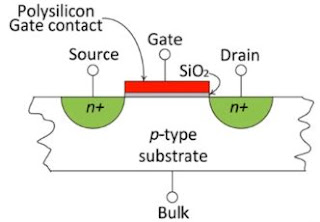FIG 1: Side/Cross-sectional view of an NMOS.

Side-section (above) of NMOS - Here, the Bulk is a p-type substrate which is a p-type semiconductor. The Source and Drain are N-Type semiconductors. The region between the Source and Drain bove the p-type substrate is called a Channel. Above this region, there is an insulator which is usually SiO2 or Silicon dioxide which is covered by a Gate (polysilicon). The Gate has a high Resistance. So basically, between our Gate and Substrate, we have our Insulator.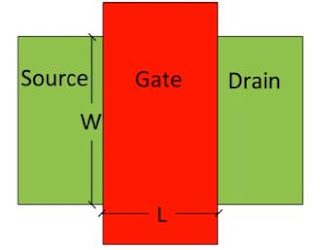Top-view of the above described NMOS.

From the top-view, you can see the Source and Drain are on the left and right side of the Gate, respectively. The width of the Gate is actually the Length (L) here, shown below. But, width which is the Width (W) of Source and Drain, cannot be shown here on 2-D side-section.NMOS Transistor Working - We connect our Gate to a Positive (+) Voltage (Gate Voltage VG). When we increase this Voltage, assuming that the Source and the Bulk are Grounded, the Channel attracts a Negative charge. The Drain is connected to a Positive (+) Voltage VD as well. Since Vis positive, the electrons move from Source to Drain. So, the direction of the Current is opposite of the direction of electron movement. Hence, the current direction is from the Drain to the Source called ID or IDS.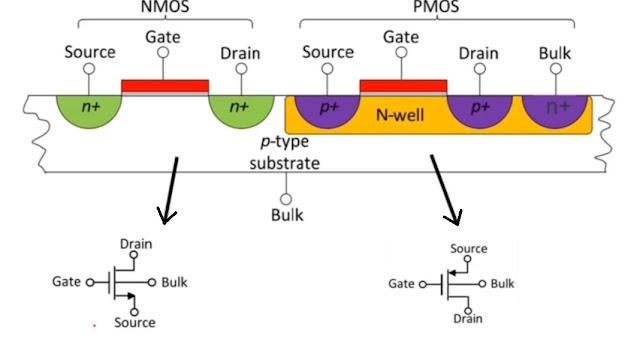NMOS and PMOS

We can create a PMOS by having an N-well inside the p-type substrate. Inside the N-well we make our PMOS. Now, the Source and the Drain are p-type semiconductors, and the Bulk is n-type. In the PMOS transistor symbol, the arrow is from the Source to the Gate. Whereas, in NMOS, it is drawn from the Gate to the Source.

NMOS Curves: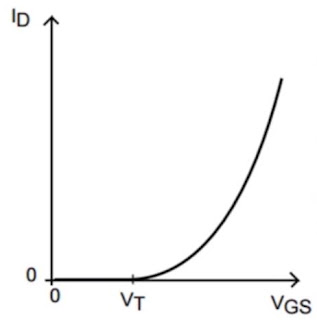We apply Gate Voltage (VGS) to the Gate and Drain Voltage (VDS which is a constant and not zero) to the Drain and ground the Source. In the beginning, the Drain has zero current and when we start increasing the VGS. When the Gate Voltage reaches a Threshold Voltage (VT), we say the Transistor is On, and when we increase the Voltage after VT, we get higher current values. Along with this, if we increase VDS in reference to the same Gate Voltage, we will get higher Drain current values.On changing the axes (swapping VDS and VGS axes), and we are changing VGS and VDS at the same time. The three operating regions of the above NMOS are: Linear or Triode/Ohmic region, Saturation region, Cut-off region.

In the Linear region, VDS < VGS - VT

At the transition (border) state, VDS = VGS - VT.

On passing the border and getting into the Saturation region, the VDS > VGS - VT.

Also, at the lower value VGS the transistor is working in the weak inversion region. It does not mean that the transistor doesn't work at the weak inversion region. It simply means that it has low VGS (lower than VT), and in fact, this property of MOS has many applications.

Current Equations:
• Saturation Region:
ID = K (W/L) (VGS - VT)2 (1 + λ VDS)

at VDS > VGS - VT and VGS ≥ VT

where, k =  1/2 μn Cox and Cox = (Ɛo . Ɛr) / tox

• W = Transistor width
• L = Transistor length
• λ = 1/V

where, VA is the early voltage which is negative (lambda is the inverse of the extrapolated point of VGS curves called V- shown below). Also, the early voltage VA is high for higher Channel Length (L). Thus for the technologies above 180nm, the "(1 + λ VDS)" part in the ID equation is as low that it can be ignored or assumed to be zero. But, in a short channel device, we can say that the Current is increasing.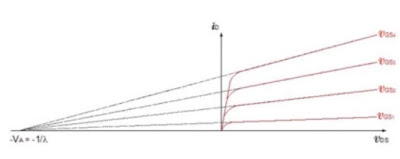• μn = Electron mobility (cm2/V.sec)
• Cox = Oxide capacitance F
• Ɛo . Ɛr = total permittivity F/cm    3.9 x 8.85 x 10-14
• tox = Oxide thickness cm, i.e. SiO2 thickness which is a very low value

• Triode Region: In this, the current depends on VGS and VDS.
I= K (W/L) ( 2(VGS - VT).VDS - V2DS )

at VDS < VGS - VT and VGS ≥ VT

*Hence, for PMOS transistors, you can derive the relation by changing VGS to VSG and VDS to VSD. Also, VSD < VSG - VT.

Following this post, the next post is a Powerful Review on ASIC Design based on the above concepts with an example (click here).